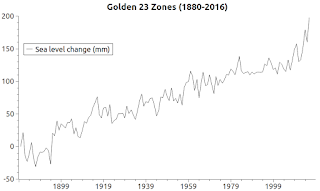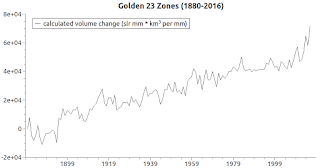## Saturday, October 7, 2017

### On The More Robust Sea Level Computation Techniques - 5Fig. 1 Determining Ocean Mass Change
I. The Critical Numbers

The most critical value to determine in thermosteric sea level rise (thermal expansion) calculations is the mass at the time the calculations commence.

That is not as difficult to determine as one might think.

In Fig. 1 the technique I use is illustrated in a manner that further illustrates what I wrote in a previous post in this series:Fig. 2
The Golden 23 numbers indicate about 197 mm (about 7.8 inches) of sea level rise during the graphed time frame.

That comports well with the NASA estimation that "sea level has risen about eight inches since the beginning of the 20th century" (NASA, emphasis added).
(On The More Robust Sea Level Computation Techniques - 4). The official global mean sea level rise during the graphed span of time is about 8 inches, which is about 200 millimeters (Fig. 1 shows 197.136 mm).

II. First Numbers FirstFig. 3
The first thing we need to know is the volume of the ocean at a given year, which I glean from “The Volume of Earth's Ocean” (Oceanography, vol. 23, no. 2, 2010, pp. 112–114; PDF version).

In 2010 that paper was published with the new calculated amount of 1,332,370,930.2 km3.

From that value, by calculation we can derive:
1,332,370,930.2 ÷ 3.6822 = 361,841,000 (cu km per km of depth)
361,841,000 ÷ 1000 = 361,841 (cu km per m of depth)
361,841 ÷ 1000 = 361.841 (cu km per mm of depth)
The sea level rise, from 1880 - 2016, shown by the Golden 23 PSMSL tide gauge stations is 197.136 millimeters.Fig. 4
Looking at my CSV file, the ocean mass in 2010 is 1,332,370,930.2 km3, the ocean mass in 2016 is 1,332,385,221.75 km3, and the ocean mass in 1880 is 1,332,313,889.78 km3.

So, 1,332,385,221.75 - 1,332,313,889.78 is 71,331.97 km3.

That is the mass increase in cubic kilometers (km3).

To double check the calculations, we multiply the sea level rise in millimeters by the cubic kilometers per millimeter of ocean: 197.136 × 361.841 = 71,331.887376 km3.

How close they are is determined by: 71,331.97 − 71,331.887376 = 0.082624 mm) close enough?

If that is close enough, then the ocean mass in 1880 was 1,332,313,889.78 km3.

That is the mass value we must use to properly calculate thermal expansion:
"A common practice in sea level research is to analyze separately theFig. 5
variability of the steric and mass components of sea level. However, there are conceptual and practical issues that have sometimes been misinterpreted, leading to erroneous and contradictory conclusions on regional sea level variability. The crucial point to be noted is that the steric component does not account for volume changes but does for volume changes per mass unit (i.e., density changes). This indicates that the steric component only represents actual volume changes when the mass of the considered water body remains constant.
"
(On The More Robust Sea Level Computation Techniques). If we do not use that value, instead using the increasing mass / volume quantity, then the thermal expansion mass value becomes about thirteen times more than all of the ocean volume increase (the variable use totals to a 910, 589 km3 thermal expansion increase, when the maximum mass / volume increase is 71,331.97 km3).

The graph at Fig. 3 shows how really way off the improper use of the formula will make the thermal expansion calculation (see Section V. here for how the TEOS toolkit is used to calculate that issue).

III. The Other Graphs

The graph at Fig. 4 shows a lot of activity in both thermal expansion and thermal contraction, as I discovered about a year and a half ago (On Thermal Expansion & Thermal Contraction).Fig. 6

The net result, though, is thermal contraction.

The graph at Fig. 5 contains both the wrong use of the formulas and the right use of the formulas.

You can clearly see that the use of the increasing ocean mass/volume vs. the use of the initial ocean mass/volume radically distorts the result.

And the graph at Fig. 6 shows the proper ocean mass / volume change increase of 71,331.97 km3 associated with the 197.136 mm sea level rise.

BTW, I do not use the abstract mass / volume values in the calculations any longer.

For the water temperature and salinity history, I use calculations that more accurately compute those values, so the 1968 beginning date for WOD water temperature and salinity measurements, and the satellite beginning date of 1993, are no longer a hindrance (due to lack of data).

IV. Conclusion

Solving the mass / volume quantities of the ocean has made the entire exercise stable now.

So, I want to get back to some of the models that I have not used in a long time, and plug the new TEOS toolkit, ocean mass / volume values, and other factors into projecting some sea level changes in various parts of the world.

The next post in this series is here, the previous post in this series is here.